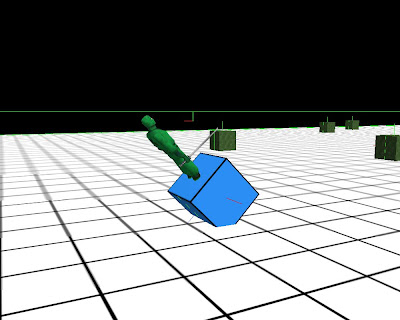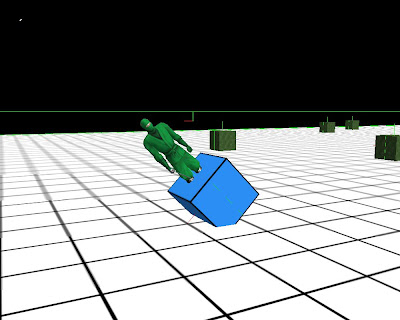## Thursday, 10 February 2011

### Developer Journal 560 degrees from up vector. Good. yaw 0, pitch 0, roll 0

2:24 PM
One of the hardest things to do is to keep going each day when you're feeling doubt and time pressures.

I tried comparing the angle between two quaternions and no luck. Quaternions could work differently to vectors in this way.

Nope. I think, I'm messing up defining an up quaternion.

4:28 PM
I hate quaternions. So much.

5:10 PM
Calculating the angle between an up vector and a direction vector only works in when I rotate in some directions.

5:30 PM
I can rotate objects as much as I like but I can't get the current rotation level back to check whether an object has rotated too far.

6:17 PM
Looking at the direction vector doesn't help. Perhaps, I can look

6:26 PM
GOT IT!
1) Create two up vectors (0, 1, 0). Call them Alpha and Bravo.
2) Rotate Bravo by the robot's orientation.
3) Calculate the angle between Alpha and Bravo.45 degrees from up vector. Good. yaw 0, pitch -45, roll 045 degrees from up vector. Good. yaw -45, pitch -90, roll 9045 degrees from up vector. Good. yaw 0, pitch, 135, roll -18045 degrees from up vector. Good. yaw 45, -90, 9045 degrees from up vector. Good. yaw 0, pitch 45, roll 0

YES! YES! YES!

I don't know why this doesn't work when Bravo is (0, 0, -1) and I don't know how to do this with quaternions.

More screen shots to illustrate why using pitch and yaw to detect the extent of tipping over was difficult.45 degrees from up vector. Good yaw 0, pitch 0, roll -45

Up till now we could use the rule that a robot has tipped too far when either absolute pitch or absolute roll has exceeded 45 degrees.46 degrees from up vector. Good. yaw -34, pitch -18, roll -34

However, there are situations like this which makes using such a rule difficult.

Some code fragments to illustrate what I'm doing.

```void Robot::Rotate( const Direction& dir ) {
const float increment = 5.0f;
float yaw = 0.0f;
float pitch = 0.0f;
float roll = 0.0f;
switch( dir ) {
case ROTATE_DOWN:
pitch = Ogre::Degree( -increment ).valueRadians();
break;
case ROTATE_UP:
pitch = Ogre::Degree( increment ).valueRadians();
break;
case ROTATE_LEFT:
yaw = Ogre::Degree( increment ).valueRadians();
break;
case ROTATE_RIGHT:
yaw = Ogre::Degree( -increment ).valueRadians();
break;
case ROLL_LEFT:
roll = Ogre::Degree( -increment ).valueRadians();
break;
case ROLL_RIGHT:
roll = Ogre::Degree( increment ).valueRadians();
break;
default:
break;
}

// get transform
btTransform transform;
transform.setIdentity();
transform = rigidBody->getCenterOfMassTransform();

// modify transform
btMatrix3x3 matrix3x3;
matrix3x3.setEulerYPR( roll, yaw, pitch );
transform.getBasis() *= matrix3x3;

// apply transform
rigidBody->setCenterOfMassTransform( transform );

// debug
transform.getBasis().getEulerYPR( roll, yaw, pitch );
cout << "yaw : " << nint( Ogre::Radian( yaw ).valueDegrees() )
<< endl;
cout << "pitch : "  << nint( Ogre::Radian( pitch ).valueDegrees() )
<< endl;
cout << "roll : "  << nint( Ogre::Radian( roll ).valueDegrees() )
<< endl;

// WORKS!
const btVector3 upVec( 0.0f, 1.0f, 0.0f );
const btQuaternion rotQua = transform.getRotation();
btVector3 rotVec( 0.0f, 1.0f, 0.0f );
rotVec = transform.getBasis() * rotVec;
const float angle = upVec.angle( rotVec );

// ARGH!
//const btQuaternion upQua( btVector3( 1.0f, 0.0f, 0.0f ), 90.0f ); // up
//const btQuaternion upQua( btVector3( 0.0f, 0.0f, 1.0f ), 0.0f ); // up
//const btQuaternion upQua( btVector3( 0.0f, 1.0f, 0.0f ), 0.0f ); // up
//btQuaternion rotQua( upQua );
//rotQua = rotQua * transform.getRotation();
//const float angle = upQua.angle( rotQua );

cout << "angle: " << Ogre::Radian( angle ).valueDegrees() << endl;
}
```

Pomodoros
1 1 1 1
1 1 1 1
1

RELATED

1.Hmm. What set of values is OGRE using to render the orientation of your object?

2.Hi Anonymous,

I took a vector representing position and a quaternion representing orientation from Bullet and then used them in Ogre. I'll post up the actual pitch, yaw, and roll values for each of the screen-shots in a few hours.

3.Hi Anonymous,

I've added a few screen shots, yaw, pitch, and roll values, and some code to clarify the difficulties I had and the approach that I found.

The function allows me to manually rotate a robot depending on the input direction.

Line 36 and 43 where I call setEulerYPR() and getEulerYPR() look a bit funny because either they don't work as they should so I had to modify how I used them or I'm way off.

The key part of this is Bullet code with a few convenience fragments from Ogre.